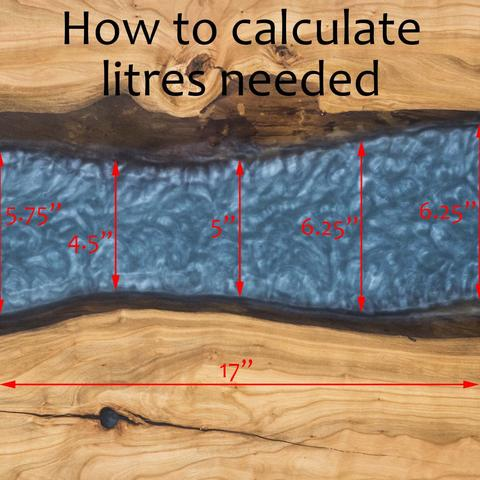# How to calculate Epoxy volume required

Posted by Bob Liu on

How to calculate Epoxy resin volume required for river table?

Use 2.4 litres Epoxy for 1 inch thick, 1 square foot volume space (or say 1.75 litres resin plus 0.65 litres hardener)

Example:

See picture below, select 5 points equally, average width of river (5.75 + 4.5 + 5 + 6.25 + 6.25) / 5 = 5.55", the river is 1.5" thick, 17" long, so totally need 5.55" x 17" x 1.5" / 144 * 2.4 = 2.36 litres